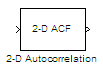# 2-D Autocorrelation

2-D autocorrelation of input matrix

• Library:
• Computer Vision Toolbox / Statistics

•## Description

The 2-D Autocorrelation block computes the 2-D autocorrelation of the input vector or matrix.

## Ports

### Input

expand all

Input array, specified as a vector or matrix.

Data Types: `single` | `double` | `int8` | `int16` | `int32` | `uint8` | `uint16` | `uint32` | `fixed point`
Complex Number Support: Yes

### Output

expand all

Autocorrelation of the input array, returned as a vector or matrix. The data type of the output is the same as that of the input.

Data Types: `single` | `double` | `int8` | `int16` | `int32` | `uint8` | `uint16` | `uint32` | `fixed point`
Complex Number Support: Yes

## Parameters

expand all

For details on fixed-point block parameters, see Specify Fixed-Point Attributes for Blocks.

Select this parameter to prevent the fixed-point tools from overriding the data types you specify in this block. For more information, see Lock the Output Data Type Setting (Fixed-Point Designer).

## Block Characteristics

 Data Types `double` | `fixed point` | `integer` | `single` Multidimensional Signals `no` Variable-Size Signals `yes`

## Algorithms

If the input Ais a vector with dimension M-by-1 then the equation for 1-D discrete autocorrelation is:

`$C\left(n\right)=\sum _{m=0}^{M-1}A\left(m\right)\ast A\left(m+n\right)$`

where $0\le n\le 2M-1$ .

The output is an autocorrelation vector of size $\left(2M-1,1\right)$.

If the input A is a matrix with dimension M-by-N then the equation for the 2-D discrete autocorrelation is:

`$C\left(i,j\right)=\sum _{m=0}^{\left(M-1\right)}\sum _{n=0}^{\left(N-1\right)}A\left(m,n\right)\cdot conj\left(A\left(m+i,n+j\right)\right)$`

where $0\le i<2M-1$ and $0\le j<2N-1$.

The dimension of the output autocorrelation matrix is $\left(2M-1,2N-1\right)$.